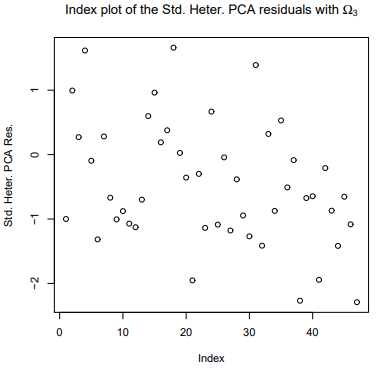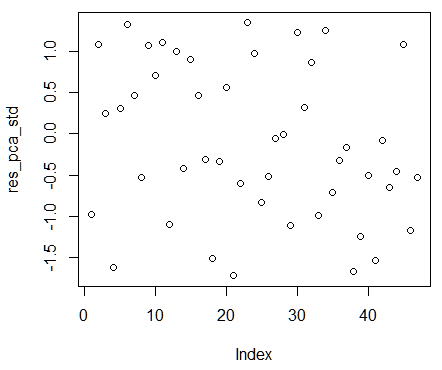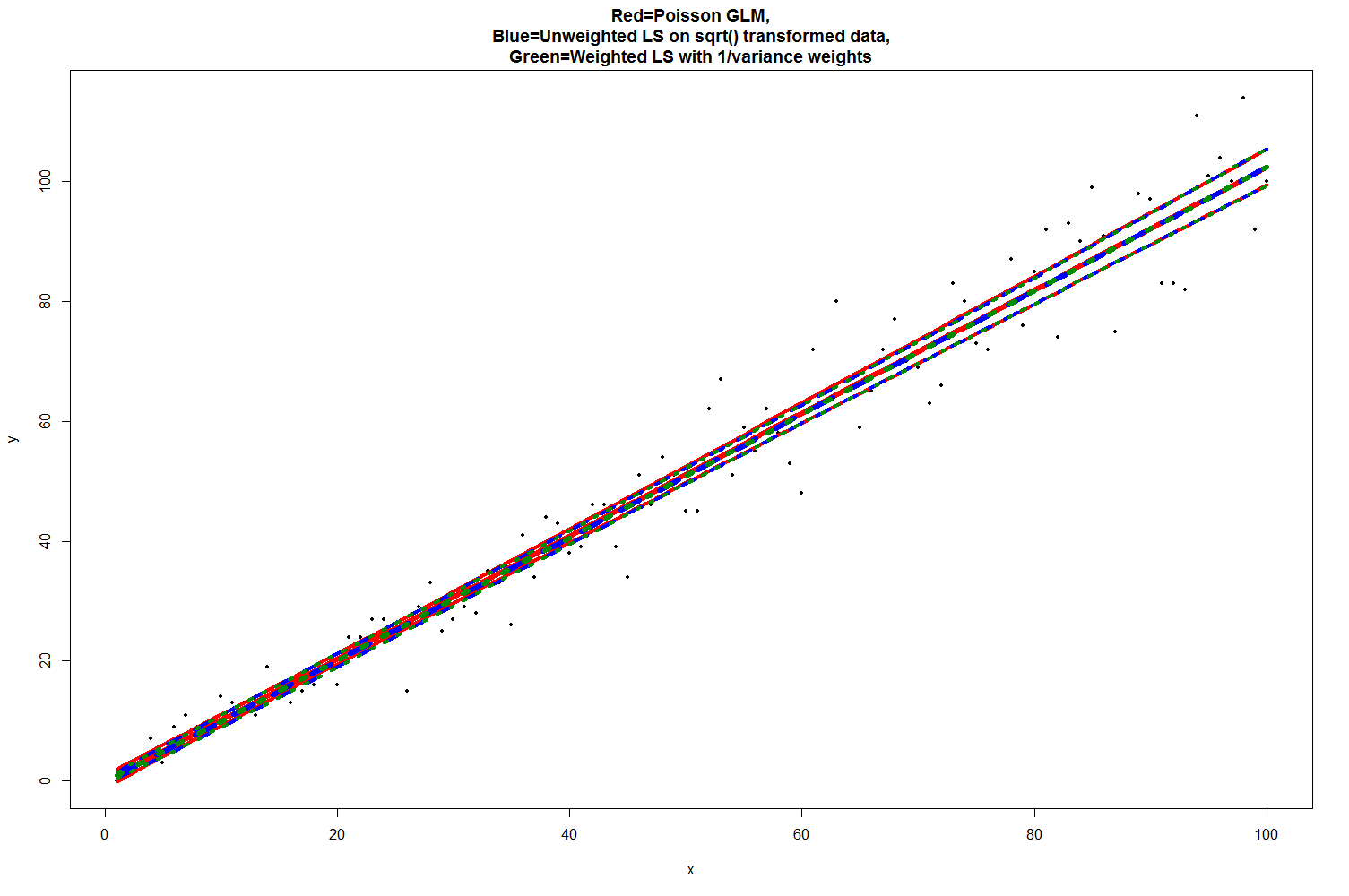## #StackBounty: #r #regression #residuals #heteroscedasticity #eigenvalues Failure to replicate calculation of PCA residuals in linear re…

### Bounty: 100

In their preprint, Rocha et al. suggest a new type of residual for linear regression models with heteroscedasticity. They call their new residual PCA residuals. I have tried to replicate some of their examples but failed. I would be very grateful if someone could give me a hint where I’m wrong.

## Background

Briefly, they assume the usual linear model of the form in matrix notation $$Y=Xbeta + epsilon$$

where $$Y = (Y_1ldots, Y_n)^T$$, $$X = (X_{ij})$$ is an $$ntimes p$$ matrix, $$beta = (beta_1,ldots, beta_p)^T$$ and $$epsilon = (epsilon_1, ldots, epsilon_n)^T$$. Further, let $$Omega = mathrm{Cov}(epsilon) = mathrm{diag}(sigma_1^2, ldots, sigma_n^2)$$ (with $$0 for $$i=1,ldots, n$$). They show that the residuals satisfy
$$hat{epsilon} = (I_n – H)ysim N_n(0, (I_n – H)Omega)$$
where $$I_n$$ is the identity matrix and $$H$$ the hat matrix with diagonal elements $$h_i$$. There exist a number of known heteroscedasticity-consistent estimators for $$Omega$$ but I will focus on $$HC_3$$ here:

$$widehat{Omega}_{3} = mathrm{diag}(1/(1-h_i)^{2})widehat{Omega}$$

where $$widehat{Omega}=mathrm{diag}(hat{epsilon}^2_{1}, ldots, hat{epsilon}^2_{n})$$.

## PCA residuals

To get the PCA residuals, perform a spectral decomposition (Eigendecomposition) of the form

$$(I_n – H)widehat{Omega}_{3} = Qwidehat{Lambda}Q^{-1}$$

where $$Q$$ is an orthogonal matrix of eigenvectors and $$Lambda = mathrm{diag}(overbrace{lambda_1, ldots, lambda_{n-p}}^{n-p}, underbrace{0, ldots, 0}_{p})$$. That means that the last $$p$$ eigenvalues are zero. Finally, the standardized PCA residuals are defined as

$$R_{i} = frac{Qhat{epsilon}}{sqrt{lambda_{i}}},quad i = 1,ldots, n-p.$$

One problem in my calculations below (I think) is that this covariance matrix $$(I_n – H)widehat{Omega}_{3}$$ is not positive and symmetric.

## An example

The authors use the `states.dta` dataset to illustrate the use of their PCA residuals. I have tried to replicate their calculations but was not able to.

Consider the multiple linear regression model
$$mathrm{csat}i = beta_0 + beta_1mathrm{percent}_i + beta_2mathrm{high}_i + beta_3mathrm{percent}{i}^2 + epsilon_i, quad i = 1,ldots, 51$$

Here are my steps in R:

``````library(foreign)

mod <- lm(csat~percent + high + I(percent^2), data = dat)
``````

Calculate the heteroscedasticity-consistent matrix $$widehat{Omega}_{3}$$:

``````res2 <- as.numeric(residuals(mod)^2)
omegahat_HC3 <- diag(res2/(1 - hatvalues(mod))^2)
``````

Now perform the spectral decomposition (note that the decomposition results in some complex numbers but I don’t know if that’s a problem):

``````X <- model.matrix(mod)
Ident <- diag(nobs(mod))
Hatmatrix <- tcrossprod(svd(X)\$u)

covmat <- (Ident - Hatmatrix)%*%omegahat_HC3

decomp <- eigen(covmat) # Spectral decomposition
Q <- decomp$$vectors # Eigenvectors Lambda <- decomp$$values # Eigenvalues
``````

Finally, calculate the PCA residuals (note that I’m multiplying the residuals with the transpose of $$Q$$, because the results where nonsensical otherwise. But I’m unsure why that’s the case):

``````res <- as.numeric(residuals(mod))

res_pca <- t(Q)%*%res # Unstandardized PCA residuals

n <- nobs(mod)
p <- length(coef(mod))

res_pca_std <- Re(res_pca[1:(n - p)]/sqrt(Lambda[1:(n - p)]))
``````

The authors show the following index plot of the PCA residualsHere is the index plot of my calculations:My PCA residuals are obviously not quite the same but I don’t know here I was going wrong with my calculations.

Can anybody explain why my calculations are off and why?

Get this bounty!!!

## #StackBounty: #r #heteroscedasticity #poisson-regression #weighted-regression Closest approximation of a Poisson GLM using weighted lea…

### Bounty: 100

I have an application where I would like to approximate a Poisson GLM with identity link, i.e. a `glm` of the form

``````fit = glm(y~x1+x2+x3+..., family=poisson(link=identity), data=data)
``````

using a (noniterative) weighted least squares model fit instead, ie using

``````fit = lm(y~x1+x2+x3+...,, weights=..., data=data)
``````

I was wondering what would be the correct/best weights to use though to take into account the mean/variance relationship?

For Poisson the variance=mean, so would that imply that I should use weights=1/variance, i.e. `weights = 1/(y+epsilon)` with small `epsilon` (e.g. 1E-10)? I would like to use weighted OLS instead of a GLM mainly for computational reasons (a `lm.fit` typically being 5-10x faster than a `glm.fit`) and also because the identity link Poisson GLM in R doesn’t seem to be implemented in a very robust way (it often complains about it not finding valid starting values). A quick test indeed shows that such a weighted LS fit indeed is very close to the corresponding Poisson GLM.

When working with a single covariate `sqrt` transforming `x` and `y` to stabilize the variance and doing a regular OLS fit on those also seems to give a nearly identical fit as the Poisson GLM. But this only works with a single covariate though.

I was wondering if both of these procedures are generally accepted approximations of Poisson GLMs? And how they would typically be referred to, and what a good citation would be for both recipes?

To enforce nonnegativity coefficients on the fitted coefficients I would then also like to use `nnls` or `glmnet` with `lower.limits=0` (as glmnet does not support identity link Poisson; h20 does but does not support nonnegativity constraints). So would using `1/variance` weights within a regular (nonnegative) weighted least squares framework be an OK thing to do to approach a Poisson error structure?

Quick test :

``````x=1:100
set.seed(1)
y=rpois(n=length(x), lambda=x)
epsilon=1E-10
weights = 1/(y+epsilon)
weights = nrow(data)*weights/sum(weights)

data=data.frame(x=x,y=y,weights=weights)
fit = glm(y~x, family=poisson(link=identity), data=data) # Poisson GLM
lmfit = lm(sqrt(y)~sqrt(x), data=data) # OLS with x & y sqrt transformed to stabilize variance
wlmfit = lm(y*sqrt(weights) ~ x*sqrt(weights), data=data) # weighted OLS with 1/variance weights
preds.glm = data.frame(ypred=preds.glm[,1],
y.lwr=preds.glm[,1]-1.96*preds.glm[,2],
y.upr=preds.glm[,1]+1.96*preds.glm[,2])
preds.lm = do.call(cbind,predict(lmfit,se.fit=TRUE)[1:2])
preds.lm = data.frame(ypred=preds.lm[,1]^2,
y.lwr=(preds.lm[,1]-1.96*preds.lm[,2])^2,
y.upr=(preds.lm[,1]+1.96*preds.lm[,2])^2)
preds.wlm = do.call(cbind,predict(wlmfit,se.fit=TRUE)[1:2])
preds.wlm = data.frame(ypred=preds.wlm[,1]^2,
y.lwr=(preds.wlm[,1]-1.96*preds.wlm[,2])^2,
y.upr=(preds.wlm[,1]+1.96*preds.wlm[,2])^2)
dev.off()
plot(x,y,pch=16,main="Red=Poisson GLM,nBlue=Unweighted LS on sqrt() transformed data,nGreen=Weighted LS with 1/variance weights",cex=0.5)
lines(x,preds.glm$$ypred, col="red",lty=1,lwd=6) lines(x,preds.glm$$y.lwr, col="red",lty=1,lwd=4)
lines(x,preds.glm$$y.upr, col="red",lty=1,lwd=4) lines(x,preds.lm$$ypred, col="blue",lty=2,lwd=6)
lines(x,preds.lm$$y.lwr, col="blue",lty=2,lwd=4) lines(x,preds.lm$$y.upr, col="blue",lty=2,lwd=4)
lines(x,preds.lm$$ypred, col="green4",lty=3,lwd=6) lines(x,preds.lm$$y.lwr, col="green4",lty=3,lwd=4)
lines(x,preds.lm\$y.upr, col="green4",lty=3,lwd=4)
``````Also relevant perhaps is this link.

Get this bounty!!!

## #StackBounty: #hypothesis-testing #heteroscedasticity #poisson-regression #overdispersion How to perform over-dispersion test where nul…

### Bounty: 50

If I understand correctly, a quasi Poisson regression assumes roughly that
$$mbox{E}left[yleft|xright.right] = exp{left(x^{top}betaright)}, quad mbox{VAR}left(yleft|xright.right) = sigma^2 exp{left(x^{top}betaright)},$$
and estimates both $$beta$$ and $$sigma^2$$. (Poisson regression further assumes $$sigma=1$$, rather than estimating it.)

I would like to test the variance assumption. That is, assuming
$$mbox{E}left[yleft|xright.right] = exp{left(x^{top}betaright)}, quad mbox{VAR}left(yleft|xright.right) = fleft(xright) exp{left(x^{top}betaright)},$$
I would like to test the null hypothesis
$$H_0: fleft(xright) = c,,,mbox{for some},c.$$

The most widely used test, as given by Cameron & Trivedi (and implemented in `AER::dispersiontest`), seems to assume
$$mbox{E}left[yleft|xright.right] = mu = exp{left(x^{top}betaright)}, quad mbox{VAR}left(yleft|xright.right) = mu + alpha gleft(muright),$$
for some specified function $$gleft(cdotright)$$ (typically a linear or quadratic function), and tests the null hypothesis
$$H_0: alpha = 0.$$
Is it possible to adapt this test for my purposes? Concretely can I, through some perverted usage of `dispersiontest`, somehow assume
$$mbox{VAR}left(yleft|xright.right) = alpha_1 mu + alpha_2 mu^2,$$
and test $$H_0: alpha_2 = 0$$ regardless of $$alpha_1$$?

(I have tried to use `dispersiontest` on a `glm` object fit with `quasipoisson` family, and get an error claiming that “only Poisson GLMs can be tested”. So some extra fiddling will be required.)

Get this bounty!!!